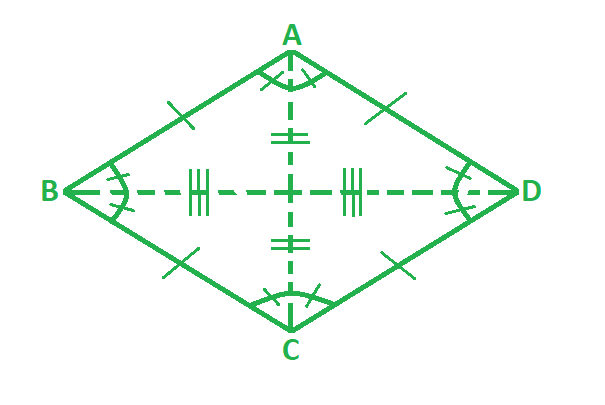# How to find the Perimeter of A Rhombus?

• Last Updated : 30 Apr, 2022

A rhombus is a parallelogram such that its four sides are equal to each other. It can be interpreted as an equilateral quadrilateral. The opposite sides of a rhombus are parallel to each other and its opposite angles are equal. The diagonals of a rhombus meet each other at 90o and equally divide the vertex angles. A square can also be termed a rhombus as it satisfies all its properties.The above figure depicts a rhombus ABCD with sides AB, BC, CD and AD of equal length and diagonals AC and BD dividing each other into two equal parts.

### Perimeter of a Rhombus Formula

The perimeter of a rhombus is equal to the sum of all its side lengths. Since all sides of a rhombus are equal to each other, its perimeter is defined as four times one of its side lengths. For example, if one side of a rhombus measures x units, its perimeter is given by 4x units.

P = 4a

where,

P is the perimeter of rhombus,

a is the side of rhombus.

### Sample Problems

Problem 1. Find the perimeter of a rhombus if its side length is 4 m.

Solution:

We have, a = 4.

Using the formula we get,

P = 4a

= 4 (4)

= 16 m

Problem 2. Find the perimeter of a rhombus if its side length is 7 m.

Solution:

We have, a = 7.

Using the formula we get,

P = 4a

= 4 (7)

= 28 m

Problem 3. Find the perimeter of a rhombus if its side length is 10 m.

Solution:

We have, a = 10.

Using the formula we get,

P = 4a

= 4 (10)

= 40 m

Problem 4. Find the perimeter of a rhombus if its side length is 12 m.

Solution:

We have, a = 12.

Using the formula we get,

P = 4a

= 4 (12)

= 48 m

Problem 5. Find the side length of a rhombus if its perimeter is 96 m.

Solution:

We have, P = 96

Using the formula we get,

P = 4a

=> a = P/4

=> a = 96/4

=> a = 24 m

Problem 6. Find the side length of a rhombus if its perimeter is 52 m.

Solution:

We have, P = 52

Using the formula we get,

P = 4a

=> a = P/4

=> a = 52/4

=> a = 13 m

Problem 7. Find the side length of a rhombus if its perimeter is 100 m.

Solution:

We have, P = 100

Using the formula we get,

P = 4a

=> a = P/4

=> a = 100/4

=> a = 25 m

My Personal Notes arrow_drop_up